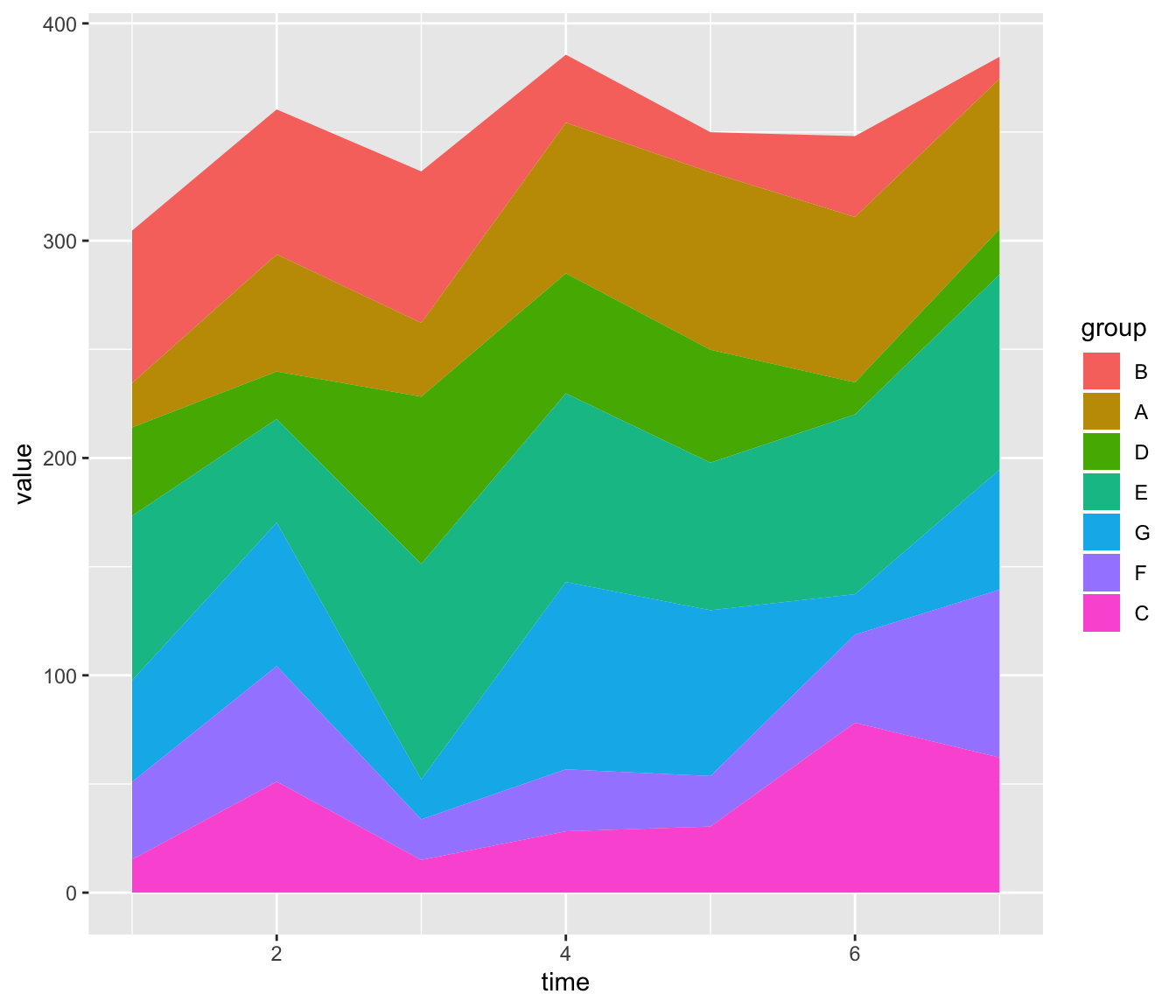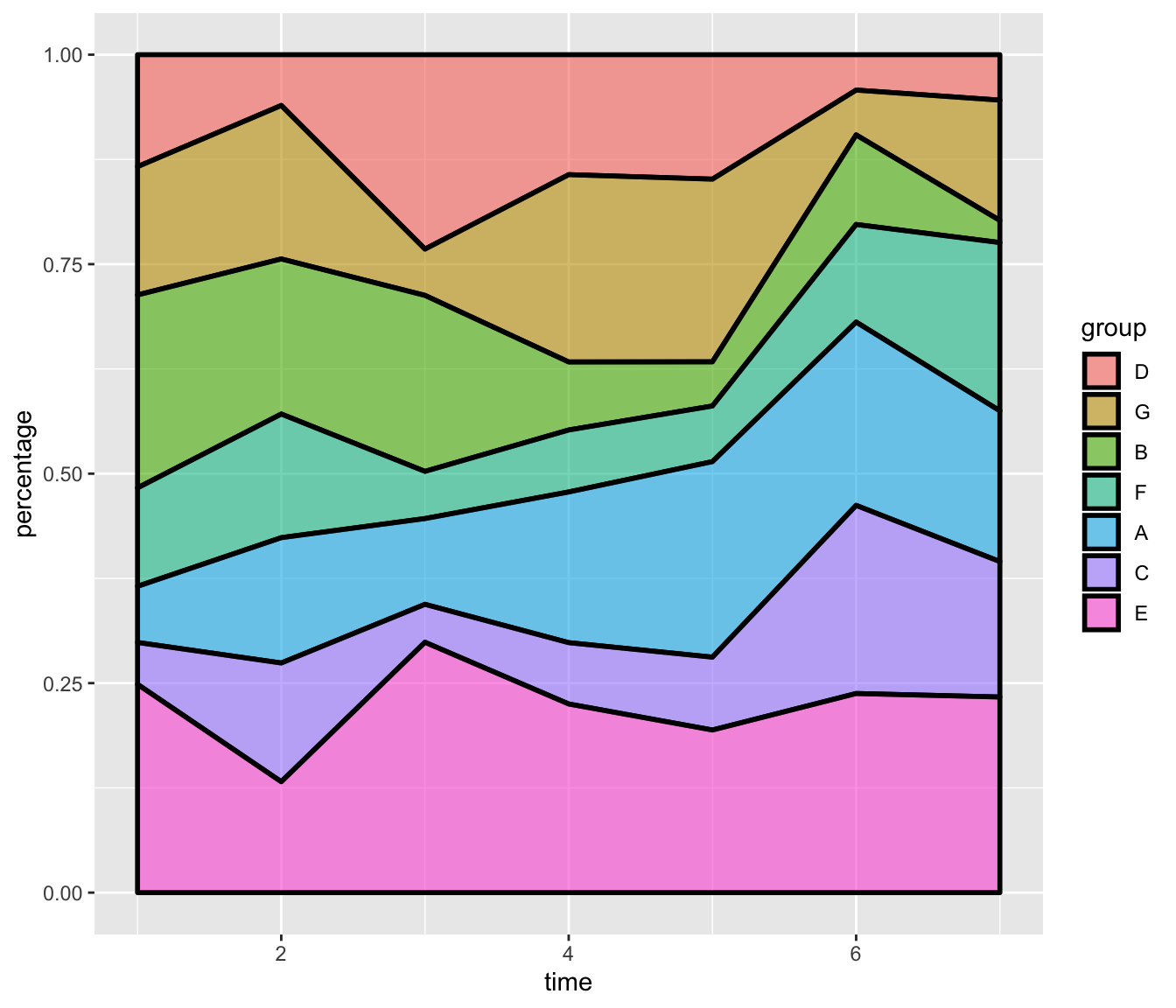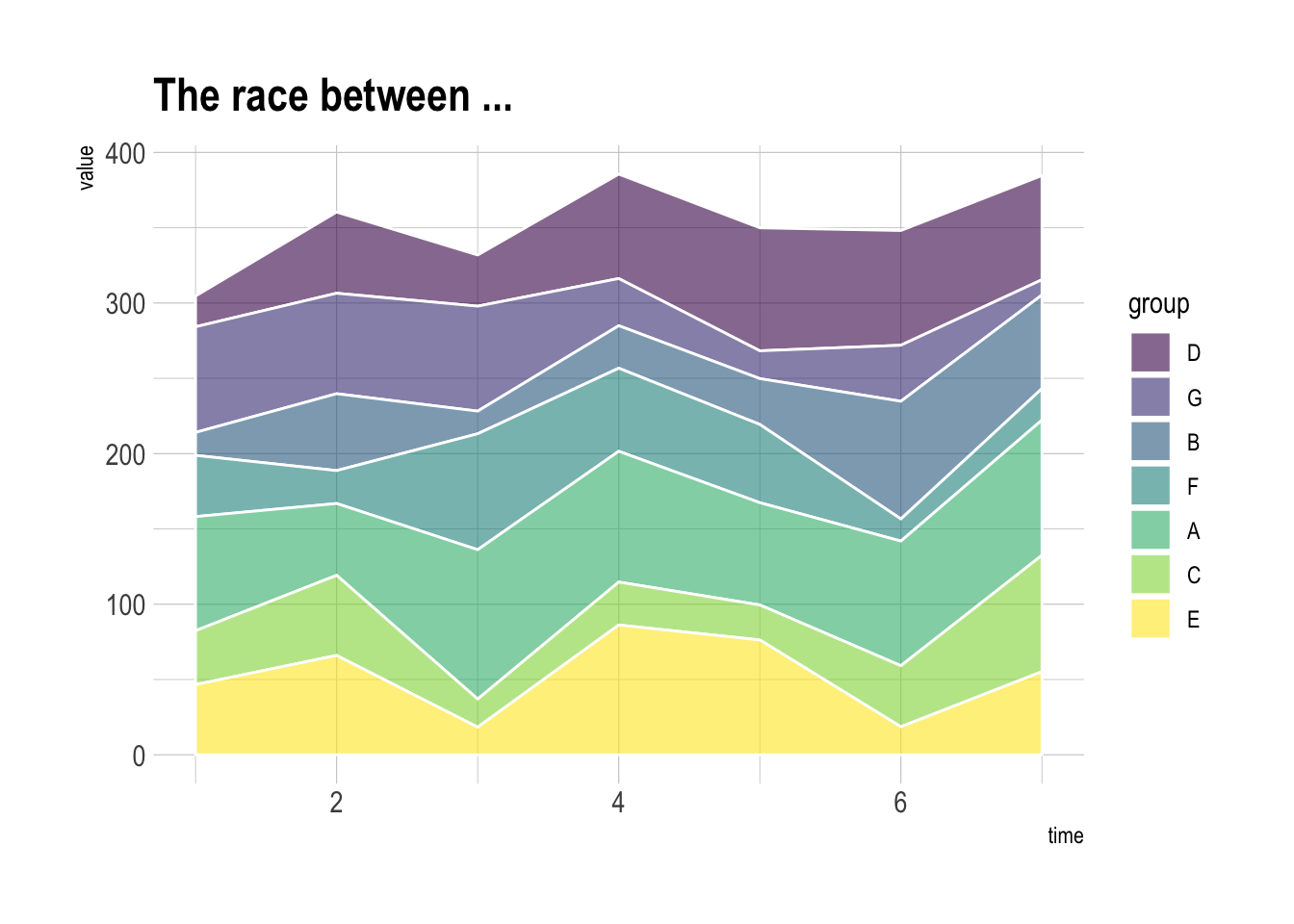# Basic Stacked area chart with R

This post provides the basics concerning stacked area chart with R and `ggplot2`. It takes into account several input format types and show how to customize the output.

# Most basic stacked area with ggplot2

The data frame used as input to build a stacked area chart requires 3 columns:

• `x`: numeric variable used for the X axis, often it is a time.
• `y`: numeric variable used for the Y axis. What are we looking at?
• `group`: one shape will be done per group.

The chart is built using the `geom_area()` function.``````# Packages
library(ggplot2)
library(dplyr)

# create data
time <- as.numeric(rep(seq(1,7),each=7))  # x Axis
value <- runif(49, 10, 100)               # y Axis
group <- rep(LETTERS[1:7],times=7)        # group, one shape per group
data <- data.frame(time, value, group)

# stacked area chart
ggplot(data, aes(x=time, y=value, fill=group)) +
geom_area()``````

# Control stacking order with `ggplot2`

The gallery offers a post dedicated to reordering with ggplot2. This step can be tricky but the code below shows how to:

• give a specific order with the `factor()` function.
• order alphabetically using `sort()`
• order following values at a specific data``````# Give a specific order:
data\$group <- factor(data\$group , levels=c("B", "A", "D", "E", "G", "F", "C") )

# Plot again
ggplot(data, aes(x=time, y=value, fill=group)) +
geom_area()

# Note: you can also sort levels alphabetically:
myLevels <- levels(data\$group)
data\$group <- factor(data\$group , levels=sort(myLevels) )

# Note: sort followinig values at time = 5
myLevels <- data %>%
filter(time==6) %>%
arrange(value)
data\$group <- factor(data\$group , levels=myLevels\$group )``````

# Proportional stacked area chart

In a proportional stacked area graph, the sum of each year is always equal to hundred and value of each group is represented through percentages.

To make it, you have to calculate these percentages first. This can be done using `dplyr` of with `base R`.``````# Compute percentages with dplyr
library(dplyr)
data <- data  %>%
group_by(time, group) %>%
summarise(n = sum(value)) %>%
mutate(percentage = n / sum(n))

# Plot
ggplot(data, aes(x=time, y=percentage, fill=group)) +
geom_area(alpha=0.6 , size=1, colour="black")

# Note: compute percentages without dplyr:
my_fun <- function(vec){
as.numeric(vec) / sum(data\$value[data\$time==vec]) *100
}
data\$percentage <- apply(data , 1 , my_fun)``````

# Color & Style

Let’s improve the chart general appearance:

• usage of the `viridis` color scale
• `theme_ipsum` of the `hrbrthemes` package
• add title with `ggtitle```````# Library
library(viridis)
library(hrbrthemes)

# Plot
ggplot(data, aes(x=time, y=value, fill=group)) +
geom_area(alpha=0.6 , size=.5, colour="white") +
scale_fill_viridis(discrete = T) +
theme_ipsum() +
ggtitle("The race between ...")``````

Related chart types

## Contact

This document is a work by Yan Holtz. Any feedback is highly encouraged. You can fill an issue on Github, drop me a message on Twitter, or send an email pasting yan.holtz.data with gmail.com.